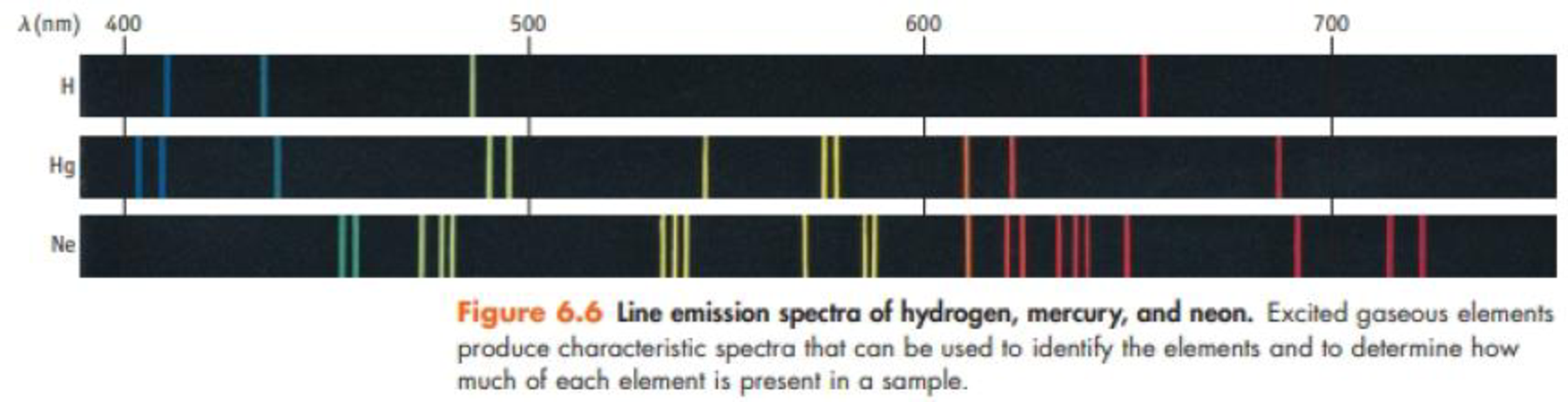Chapter 6, Problem 14PS

Chapter
Section
Textbook Problem

The most prominent line in the spectrum of neon is found at 865.438 nm. Other lines are located at 837.761 nm, 878.062 nm, 878.375 nm, and 885.387 nm.(a) In what region of the electromagnetic spectrum are these lines found?(b) Are any of these lines found in the spectrum nein shown in Figure 6.6?(c) which of these lines represents the most energetic radiation?(d) What is the frequency of the most prominent line? What is the energy of one photon with this wavelength?(a)

Interpretation Introduction

Interpretation: The region of lines in emission spectrum of neon has to be identified.

Concept introduction:

• Electromagnetic radiations are a type of energy surrounding us. They are of different types like radio waves, IR, UV, X-ray etc.
• Infrared radiation in the wavelength of 700nm to 1mm
Explanation

Emission spectrum of neon has five lines in 865.438nm, 837.761nm, 878.062nm, 878.375nm and 885.387nm where 865

(b)

Interpretation Introduction

Interpretation: Any of the given lines is whether found in the emission spectrum of neon in figure 6.6 has to be determined.

Concept introduction:

• Electromagnetic radiations are a type of energy surrounding us. They are of different types like radio waves, IR, UV, X-ray etc.
• Emission spectrum: It is a spectrum of a compound due to the electromagnetic radiation emitted when the transition of an atom or molecule from the higher to lower state.

(c)

Interpretation Introduction

Interpretation: The most energetic line among the given lines in the emission spectrum of neon has to be identified.

Concept introduction:

• Planck’s equation,

E==hcλwhere, E=energyh=Planck'sconstantν=frequency

The energy increases as the wavelength of the light decrease. Also the energy increases as the frequency of the light increases.

• The frequency of the light is inversely proportional to its wavelength.

ν=cλwhere, c=speedoflightν=frequencyλ=wavelength

(d)

Interpretation Introduction

Interpretation: The frequency and the energy per photon of most energetic line in the emission spectrum of neon have to be identified.

Concept introduction:

• Planck’s equation,

E==hcλwhere, E=energyh=Planck'sconstantν=frequency

The energy increases as the wavelength of the light decrease. Also the energy increases as the frequency of the light increases.

• The frequency of the light is inversely proportional to its wavelength.

ν=cλwhere, c=speedoflightν=frequencyλ=wavelength

Still sussing out bartleby?

Check out a sample textbook solution.

See a sample solution

The Solution to Your Study Problems

Bartleby provides explanations to thousands of textbook problems written by our experts, many with advanced degrees!

Get Started